Refer to our Texas Go Math Grade 7 Answer Key Pdf to score good marks in the exams. Test yourself by practicing the problems from Texas Go Math Grade 7 Unit 5 Study Guide Review Answer Key.

Applications of Geometry Concepts

Essential Question
How can you apply geometry concepts to solve real-world problems?

Example 1
Find (a) the value of x and (b) the measure of ∠APY.
a. ∠XPB and ∠YPB are supplementary.
3x + 78° = 180°
3x = 102°
x = 34°b. ∠APY and ∠XPB are vertical angles,
m∠APY = m∠XPB = 3x = 102°

Example 2
Find the area of the composite figure. It consists of a semicircle and a rectangle.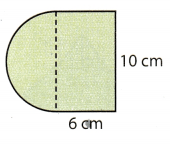Area of semicircle = o.5(πr2)
≈ 0.5(3.14)25
≈ 39.25 cm2
Area of rectangle = lw
= 10(6)
= 60 cm2
The area of the composite figure is approximately 99.25 square centimeters.

Question 1.
Find the value of y and the measure of ∠YPS (Lesson 9.1)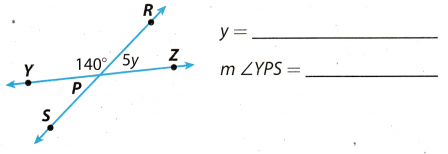Angle on the straight line SPR will be 180° and the ∠YPS and YPR will be supplementary to each other so, the
sum of both angle will be 180°.
∠YPS + ∠YPR = 180°
∠YPS + 140° = 180
∠YPS = 180 – 140
∠YPS = 40°
Also ∠YPS and ∠RPZ are vertically opposite angle so both the angle will be equal in measure.
∠RPZ = ∠YPS
5y = 40°
y = $$\frac{40}{5}$$
y = 8°
∠YPS = 40°

Find the circumference and area of each circle. Round to the nearest hundredth. (Lessons 9.2, 9.3)

Unit 5 End of Unit Assessment Answer Key Grade 7 Question 2.Given diameter of the circle = 22 inch
Circumference of circle with diameter “D” is = πD
Circumference of circle = 22 . π
= 22 × 3.14 (π = 3.14)
≈ 69 inch
Area of circle with diameter “D” is = $$\frac{\pi D^{2}}{4}$$
Area of circle = $$\frac{\pi \cdot 22^{2}}{4}$$
= $$\frac{3.14 \times 484}{4}$$
≈ 380 inch2
Circumference is 69 inch and area is 380 inch2

Question 3.Given radius of the circle = 4.5 m
Circumference of circle with radius “r” is = 2πr
Circumference of circle = 2π × 4.5
= 9 × 3.14 (π = 3.14)
= 28.26 m
Area of circle with radius “r” is = πr2
Area of circle = π × 452
= 3.24 × 20.25 (π = 3.14)
= 65.61 m2
Circumference is 28.26 m and area is 65.61 m2.

Find the area of each composite figure. Round to the nearest hundredth if necessary. (Lesson 9.4)

Question 4.Side length of the square = 9 in
Height of the triangle = 9 in
Base of the triangle = 13 – 9 = 4 in
Area of the composite figure will be the sum of area of square and the triangle.
Area of square = side2
= 92 in2
= 81 in2

Area of triangle = $$\frac{1}{2}$$ × Base × Height
= $$\frac{1}{2}$$ × 4 × 9 in2
= 18 in2
Area of composite figure = Sum of area of square and triangle
= 81 + 18 in2
= 99 in2
Hence, area of the composite figure will be 99 in2.
Area of the composite figure will be 99 in2

Question 5.Length ot the rectangle = 20 cm
Width of the rectangle = 16 cm
Radius of the semi circle = $$\frac{16}{2}$$ = 8 cm
Area of the composite figure will be the sum of area of rectangle and the semi circle.
Area of rectangle = Length × width
= 16 × 20 in2
= 320 in2Area of composite figure = Sum of area of rectangle and semi circle
= 320 + 100.18 cm2
≈ 420 cm2
Hence, area of the composite figure will be 420 cm2.

Volume and Surface Area

Example
The height of the figure whose net is shown is 8 feet. Identify the figure. Then find its volume, lateral area, and total surface area.
The figure is a rectangular pyramid.The volume of the rectangular pyramid is 384 cubic feet, the lateral surface area is 192 square feet, and the total
surface area is 336 square feet.

Exercises

Identify the figure represented by the net. Then find its lateral area and total surface area. (Less0n 10.3)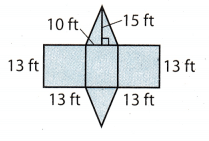The figure represented by the net is a triangular prism.
Lateral area:
One 13 ft. by 10 ft rectangle;
Two 13 ft by 13 ft. rectangles.
1 . (13 . 10) = 130
2 . (13 . 13) = 338
Lateral area: 130 + 338 = 468 ft2.
The base has the shape of triangLe with lenght of 10 ft and height of 15 ft.
Base area:
2 . ($$\frac{1}{2}$$ . 10 . 15) = 150 ft2
The total surface area is 468 + 150 = 618 ft2.

Lateral area: 468 ft2.
The total surface area is 618 ft2.

Find the volume of each figure. (Lessons 10.1,10.2)

Question 2.l = 7 in Length of base
w = 5 in Width of base
h = 12 in Height of a prism
Find the area of the base B. A prism has a base that is rectanguar, so use the formula:
B = l . w = 7 . 5 = 35 in2
Use the formula for the volume of a rectangular prism.
V = B . h = 35 . 12 = 420 in3
The volume of a rectangular prism is 420 in3.

Question 3.A pyramid has a base that is right triangle.
a = 4 m Length of one side of the triangle
b = 6 m Length of other side of the triangle
h = 9m Heightofapyramid
Find the area of the base B.Use the formula.
V = B . h = 12 . 9 = 108 m3

The volume of a triangular pyramid is V = 108 m3.

Question 4.
The volume of a rectangular pyramid is 1.32 cubic inches. The height of the pyramid is 1.1 inches and the length of the base is 1.2 inches. Find the width of the base of the pyramid. (Lesson 10.1) _____
V = 1.32 in3 The volume of a rectangular pyramid
h = 1.1 in Length of pyramid
1 = 1.2 in Length of base
w = ? Width of base

Use the formula for the volume of a pyramid to find a width of the base.
V = $$\frac{1}{3}$$ . l . w . h
1.32 = $$\frac{1}{3}$$ . (1.2) . w . (1.1)
1.32 = $$\frac{1}{3}$$ . (1.32) . w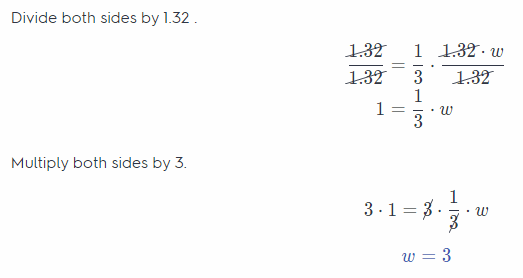The width of the base of the pyramid is w = 3 in

Mathematics Grade 7 Unit 5 Test Study Guide Answer Key Question 5.
The volume of a triangular prism is 264 cubic feet. The area of the base of the prism is 48 square feet. Find the height of the prism.
(Lesson 10.2) ____
V = B . h
B = 48 ft2 Base of prism
h =? Height of a prism
V = 264 ft3 The volume of a prism
Use the formula for the volume of a prism to find the height of the prism.
V = B . h
264 = 48 . h
Divide both sides by 48.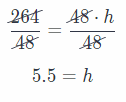The height of the prism is h = 5.5 ft.

Question 1.
Careers In Math Product Design Engineer Miranda is a product design engineer working for a sporting goods company. She designs a tent in the shape of a triangular prism. The dimensions of the tent are shown in the diagram.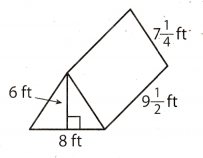a. Draw a net of the triangular prism and label the dimensions.The net of the given tent is a triangular prism.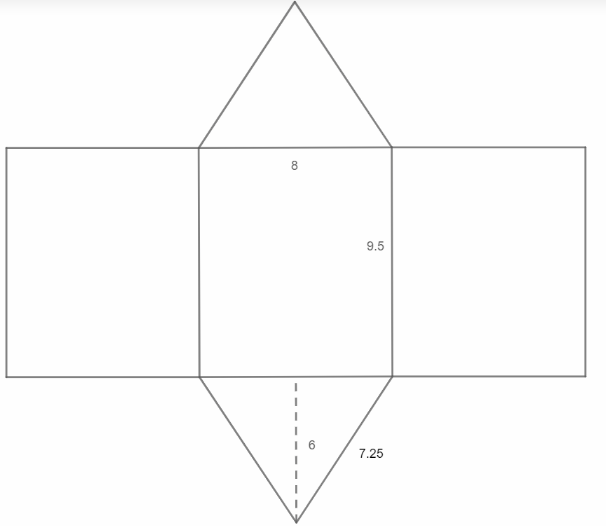b. How many square feet of material does Miranda need to make the tent (including the floor)? Show your work.
Determine the surface area of the tent or the triangular prism:
SA = 3R + 2T
SA = 3(9.5 .8) + 2 ($$\frac{4 \cdot 6}{2}$$)
SA = 3(76) + 2(12)
SA = 228 + 24
SA = 252 ft2

c. What is the volume of the tent? Show your work.
Determine the volume of the tent or triangular prism.
V = B . h
= $$\frac{4 \cdot 6}{2}$$ . 5
= 12 . 9.5
= 114 ft3

d. Suppose Miranda wants to increase the volume of the tent by 10%. The specifications for the height (6 feet) and the width (8 feet) must stay the same. How can Miranda meet this new requirement? Explain.
The new volume of the tent is:
1.1V = 1.1 . 114 = 125.4 ft3.
The length of the tent, or the height of the prism h is the parameter being changed, so:
V = B . h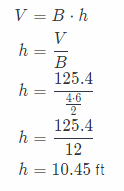The tent will get longer by 10.45 – 9.5 = 0.95 ft.

Li is making a stand to display a sculpture made in art class. The stand will be 45 centimeters wide, 25 centimeters long, and 1.2 meters high.

a. What is the volume of the stand? Write your answer in cubic centimeters.
The stand is in the form of a rectangular prism, whose volume is calculated by
V = length . width . height
Since 1.2 m = 120 cm, the volume of the stand is
V = l . w . h Write the formula for the volume
V = 45. 25. 120 Substitute the values
V = 135,000 cm3 Multiply the values

b. Li needs to fill the stand with sand so that it is heavy and stable. Each piece of wood is 1 centimeter thick. The boards are put together as shown in the figure, which is not drawn to scale. How many cubic centimeters of sand does she need to fill the stand? Explain how you found your answer.Determine the volume of the interior of the stand. To obtain that we have to “remove” 2 cm from each dimension,
thus length will be 43 cm, width is 23 cm and height is 118 cm.
V = l . w . h Write the formula for the volume
V = 43 . 23 . 118 Substitute the new values
V = 116, 702 cm3 Multiply the values

Texas Go Math Grade 7 Unit 5 Mixed Review Texas Test Prep Answer Key

Selected Response

Question 1.
The dimensions of the pyramid are given in centimeters (cm). What is the volume of the pyramid?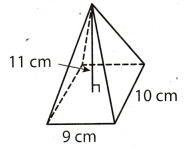(A) 222.5 cubic centimeters
(B) 330 cubic centimeters
(C) 445 cubic centimeters
(D) 990 cubic centimeters
(B) 330 cubic centimeters

Explanation:
h = 11 cm Height of pyramid
l = 9 cm Length of base
w = 10 cm Width of base
Use the formula for the voLume of a rectangular pyramid
V = $$\frac{1}{3}$$ . l . w . h
= $$\frac{1}{3}$$ . 9 . 10 . 11
= $$\frac{1}{3}$$ . 990
= 330 cm3
The volume of the pyramid is 330 cm3

Question 2.
The volume of a triangular pyramid is 437 cubic units. The height of the pyramid is 23 units. What is the area of the base of the pyramid?
(A) 6.3 square units
(B) 19 square units
(C) 38 square units
(D) 57 square units
(D) 57 square units

Explanation:
Use formula for the volume of a triangular pyramid.
B = ? Base of pyramid
h = 23units Height of a pyramid
V = 437 cubic units The volume of a pyramid
Use the formula for the volume of a pyramid to find a base area of the pyramid.
V = $$\frac{1}{3}$$ . B . h
437 = $$\frac{1}{3}$$ . B . 23
437 = (7.66) . B
Divide both sides by 7.66.The area of the base of a pyramid is 57 square units.

Hot Tip!
Make sure you look at all the answer choices before making your decision. Try substituting each answer choice into the problem if you are unsure of the answer.

Question 3.
What is the lateral surface area of the square pyramid whose net is shown?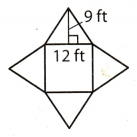(A) 216 square feet
(B) 388 square feet
(C) 568 square feet
(D) 776 square feet
(A) 216 square feet

Explanation:
Lateral surface area of square pyramid will be the sum of areas of all the triangles except base in the given figure.
Base of the triangle = 12 ft
Height of the triangle = 9 ft
We know that area of the rectangle is = $$\frac{1}{2}$$ × Base × Height
Area of triangle = $$\frac{1}{2}$$ × 12 × 9
= 54 ft2
Lateral surface area of pyramid = 4 × Area of triangle
= 4 × 54
= 216 ft2
Hence, lateral surface area is 216 ft2 and option A is correct answer

Question 4.
A one-topping pizza costs $15.00. Each additional topping costs$1.25. Let x be the number of additional toppings. You have $20 to spend. Which equation can you solve to find the number of additional toppings you can get on your pizza? (A) 15x + 1.25 = 20 (B) 1.25x + 15 = 20 (C) 15x – 1.25 = 20 (D) 1.25x – 15 = 20 Answer: (B) 1.25x + 15 = 20 Explanation: Given the cost of one-topping pizza =$13.00
The cost of additional topping on pizza = $1.25 It is given we have to spend only$20 on pizza. For “x” the number of additional topping cost will be 1.25 × x and the cost of one topping pizza will be $13. So the total cost of pizza will be the sum of cost. of one-topping pizza and the additional topping cost which will be equal to$20.
In form of equation: 1.25x + 15 = 20
Hence, option B is the correct answer.

Unit 5 Test Study Guide Geometry Basics Answer Key Question 5.
A bank offers a home improvement loan with simple interest at an annual rate of 12%. J.T. borrows $14,000 over a period of 3 years. How much will he pay back altogether? (A)$15,680
(B) $17,360 (C)$19,040
(D) $20,720 Answer: (C)$19,040

Explanation:
The principal amount of home loan (P) = $14. 000 Rate of the simple interest annually (r) = 12% Duration for which loan is taken (t) = 3 years We know that simple interest = $$\frac{P \times r \times t}{100}$$ Simple interest = $$\frac{14,000 \times 12 \times 3}{100}$$ = 140 × 36 =$5,040
Total which has to be paid = Principal Simple interest
= 14,000 + 5,040
= \$19,040
Hence, option C is correct answer.

Question 6.
What is the volume of a triangular prism that is 75 centimeters long and that has a base with an area of 30 square centimeters?
(A) 2.5 cubic centimeters
(B) 750 cubic centimeters
(C) 1,125 cubic centimeters
(D) 2,250 cubic centimeters
(D) 2,250 cubic centimeters

Explanation:
B = 30 cm2 Base of prism
h = 75 cm Height of a prism
Use the formula for the volume of the prism.
V = B . h
= 30 . 75
= 2250 cm3

The volume of a triangular prism is 2250 cubic centimeters.

Question 7.
The radius of the circle is given in meters. What is the circumference of the circle? Use 3.14 for π.(A) 25.12 meters
(B) 50.24 meters
(C) 200.96 meters
(D) 803.84 meters
(B) 50.24 meters

Explanation:
Given radius of the circle (r) = 8 m
We know that circumference of circle of radius “r” is = 2πr
Circumference of circle = 2π × 8
= 16π
(π = 3.14)
= 16 × 3.14
= 50.24 meters.
hence, option B is correct answer.

Question 8.
The dimensions of the figure are given in millimeters. What is the area of the two-dimensional figure?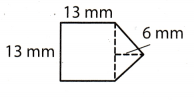(A) 39 square millimeters
(B) 169 square millimeters
(C) 208 square millimeters
(D) 247 square millimeters
(C) 208 square millimeters

Explanation:
The area of the two-dimensional figure has one 13mm. by 13 mm. square and one triangle with length of 13 mm. and height of 6 mm.
The area of the two-dimensional figure is:
(13 . 13) + ($$\frac{1}{2}$$ . 13 . 6) = 169 + 39 = 208 mm2

The area of the two-dimensional figure is 208 square millimeters.

Gridded Response

Question 9.
What is the measure in degrees of an angle that is supplementary to a 74° angle?Supplementary angle : supplementary angle means the pair of angle whose sum is 180°.
Given angle in problem: = 74°
Let the other supplementary angle be = x°
x° + 74° = 180°
x = 180° – 74°
r = 106°
Hence, other supplementary angle wilt be 106°
Steps to plot the given box are:
In 1st column : mark ‘+’ sign
In 2nd column : mark “0”
In 3rd column : mark “1”
In 4th column : mark “0”
In 5th column : mark “6”
In 6th column: mark “0”
In 7th column : mark “0”
106°

Question 10.
What is the volume in cubic centimeters of a rectangular prism that has a length of 6.2 centimeters, a width of 3.5 centimeters, and a height of 10 centimeters?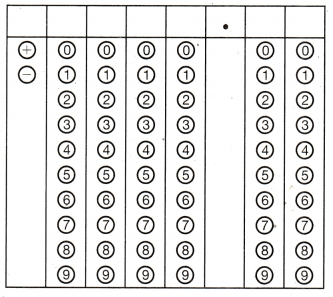Given length of the rectangular prism = 6.2 cm
Given width of the rectangular prism = 3.5 cm
Given height of the rectangular prism = 10 cm
Volume of rectangular prism = length × width × height
Volume of rectangular prism = 6.2 × 3.5 × 10
= 6.2 × 35
= 217 cm3
Hence, volume of the rectangular prism is 217 cm3.

Steps to plot the given box are:
In 1st column : mark ‘+’ sign
In 2nd column : mark “0”
In 3rd column : mark “2”
In 4th column : mark “1”
In 5th column : mark “7”
In 6th column: mark “0”
In 7th column : mark “0”
volume of the rectangular prism is 217 cm3.

Hot Tip!
It is helpful to draw or redraw a figure. Answers to geometry problems may become clearer as you redraw the figure.

What is the area of the circle in square meters? Use 3.14 for π.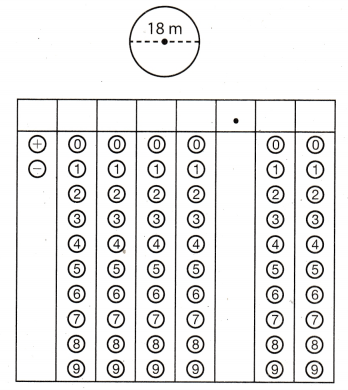Given diameter of the circle (D) = 18 m
We know that area of circle of diameter “D” is = $$\frac{\pi D^{2}}{4}$$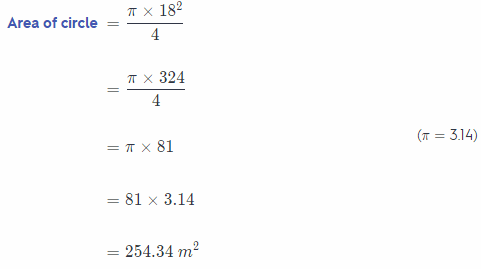hence, option B is the correct answer.

Steps to plot the given box are:
In 1st column : mark ‘+’ sign
In 2nd column : mark “0”
In 3rd column : mark “2”
In 4th column : mark “5”
In 5th column : mark “4”
In 6th column: mark “3”
In 7th column : mark “4”
Area of circle is 254.34 m2

Use the puzzle to preview key vocabulary from this unit. Unscramble the circled letters to answer the riddle at the bottom of the page.

Question 1.
NEONGTURC LANSEG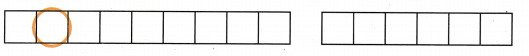Question 2.
LEOTECRAYMPMN SEGLAN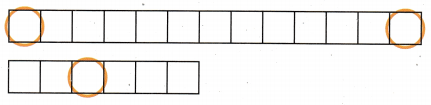Question 3.
RIMEUCEEFNRCC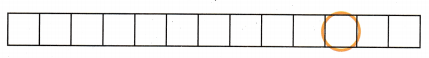Question 4.
LEARATL ERAA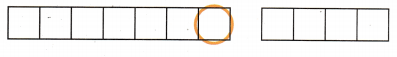Question 5.
PIECOTMOS GUISEFR